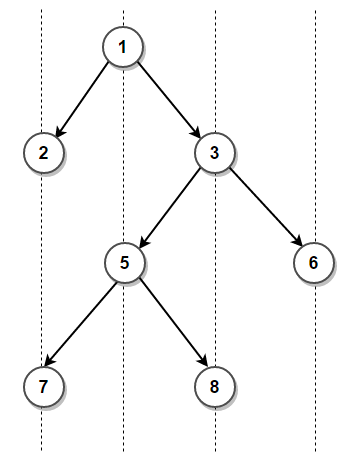# Perform vertical traversal of a binary tree

Given a binary tree, perform vertical traversal of it. In vertical traversal, we print nodes of a binary tree in vertical order by assuming that the left and right child of a node makes 45 degree angle with the parent.

For example, vertical traversal of below binary tree is

2, 7
1, 5
3, 8
6This problem can be easily solved with the help of Hashing. This post provides an overview of some of the available alternatives to accomplish this using hashing.

### 1. Using Preorder Traversal

The idea is to create an empty map where each key represents the relative horizontal distance of a node from the root node and value in the map maintains all nodes present at same horizontal distance. Then we do a pre-order traversal of the tree and we update the map. For each node, we recurse for its left subtree by decreasing horizontal distance by 1 and recurse for right subtree by increasing horizontal distance by 1. For above binary tree, the final values in the map will be

(horizontal distance -> Nodes)

-1 -> [2, 7]
0 -> [1, 5]
1 -> [3, 8]
2 -> 

Here’s a C++ program that demonstrates it:

Output:

2 7
1 5 9
3 8
10 6

The time complexity of above solution is O(nlog(n)) and auxiliary space used by the program is O(n).

### 2. Using Level Order Traversal

Since above solution uses preorder traversal to traverse the tree, the nodes might not get processed in same order as they appear in the binary tree from top to bottom. For instance, node 10 is printed before node 6 in above solution.

We can perform level order traversal to ensure the nodes are processed in same order as they appear in the binary tree. The idea remains the same as previous approach where we create an empty map whose each key represents the relative horizontal distance of a node from the root node and value in the map maintains all nodes present at same horizontal distance. The only difference is we traverse the binary tree using level order traversal instead of the pre-order traversal.

Output:

2 7
1 5 9
3 8
6 10

The time complexity of above solution is O(nlog(n)) and auxiliary space used by the program is O(n).

Exercise: Reduce time complexity to linear using std::unordered_map or std::unordered_multimap.(1 votes, average: 5.00 out of 5)Loading...

Please use our online compiler to post code in comments. To contribute, get in touch with us.
Like us? Please spread the word and help us grow. Happy coding 🙂